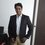# The meaning of pressure

We know from the molecular theory of the ideal gas that in an enclosed container pressure is interpreted as the momentum transfer from the gas molecules in due of its collision with the walls. The molecules will move faster if the kinetic energy of those is increased by increasing temperature. In a way pressure is also an energy density that is the average kinetic energy contained of the gas molecules divided by the total volume enclosed in the container. This is just another way to state the equation of state for an ideal gas.

However when we study the Navier Stokes Equations in the fluid mechanics and the incompressibility assumption is made, the pressure P there is defined as the static pressure. We associate the static pressure at a point in the fluid flow field as the mass of the fluid lying above that (commonly known as hydrostatic approximation).

Now I think we run into two different definitions of the pressure one from molecular gas theory and other from continuum theory of the fluid flow. If the fluid is taken as the continuum then ofcourse pressure is not simply the momentum transfer due to the molecular collisions with the wall as in the enclosure example. But again it is difficult to imagine, a variable can have two different definitions at the same time.

My question is "How exactly do we reconcile these two views of the pressure, one from molecular gas theory and another from continuum fluid mechanics?"Note by Subharthi Chowdhuri
4 years, 6 months ago

This discussion board is a place to discuss our Daily Challenges and the math and science related to those challenges. Explanations are more than just a solution — they should explain the steps and thinking strategies that you used to obtain the solution. Comments should further the discussion of math and science.

When posting on Brilliant:

• Use the emojis to react to an explanation, whether you're congratulating a job well done , or just really confused .
• Ask specific questions about the challenge or the steps in somebody's explanation. Well-posed questions can add a lot to the discussion, but posting "I don't understand!" doesn't help anyone.
• Try to contribute something new to the discussion, whether it is an extension, generalization or other idea related to the challenge.
• Stay on topic — we're all here to learn more about math and science, not to hear about your favorite get-rich-quick scheme or current world events.

MarkdownAppears as
*italics* or _italics_ italics
**bold** or __bold__ bold
- bulleted- list
• bulleted
• list
1. numbered2. list
1. numbered
2. list
Note: you must add a full line of space before and after lists for them to show up correctly
paragraph 1paragraph 2

paragraph 1

paragraph 2

[example link](https://brilliant.org)example link
> This is a quote
This is a quote
    # I indented these lines
# 4 spaces, and now they show
# up as a code block.

print "hello world"
# I indented these lines
# 4 spaces, and now they show
# up as a code block.

print "hello world"
MathAppears as
Remember to wrap math in $$ ... $$ or $ ... $ to ensure proper formatting.
2 \times 3 $2 \times 3$
2^{34} $2^{34}$
a_{i-1} $a_{i-1}$
\frac{2}{3} $\frac{2}{3}$
\sqrt{2} $\sqrt{2}$
\sum_{i=1}^3 $\sum_{i=1}^3$
\sin \theta $\sin \theta$
\boxed{123} $\boxed{123}$

## Comments

Sort by:

Top Newest

Continue fluid mechanics

- 4 years, 5 months ago

Log in to reply

×

Problem Loading...

Note Loading...

Set Loading...